Custom SearchPOWER OF A FUNCTION The integral of a function raised to a power is found by the following steps: 1. Increase the power of the function by 1. 2. Divide the result of step 1 by this increased power. 3. Add the constant of integration. Formula.PROOF: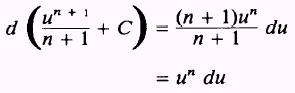Therefore,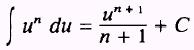NOTE: Recall that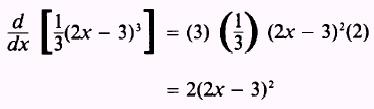EXAMPLE: Evaluate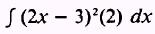SOL UTION: Let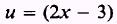so thator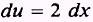Then by substitution,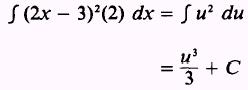Therefore,When you use this formula the integral must contain precisely du. If the required constant in du is not present, it must be placed in the integral and then compensation must be made. EXAMPLE: Evaluate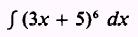SOL UTION: Let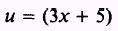so that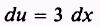We find dx in the integral but not 3 dx. A 3 must be included in the integral to fulfill the requirements of du. In words, this means the integralneeds du so that the formula may be used. Therefore, we write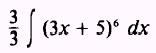and recalling that a constant may be carried across the integral sign, we write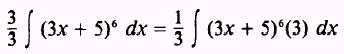Notice that we needed 3 in the integral for du, and we included 3 in the integral; we then compensated for the 3 by multiplying the integral by 1/3. Then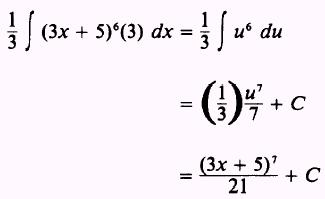EXAMPLE: Evaluate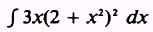SOL UTION: Let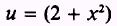so that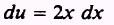Then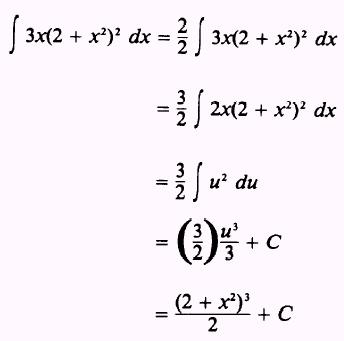PRACTICE PROBLEMS: Evaluate the following integrals: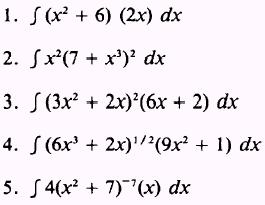ANSWERS: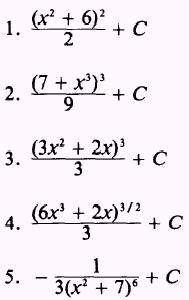Integrated Publishing, Inc. - A (SDVOSB) Service Disabled Veteran Owned Small Business